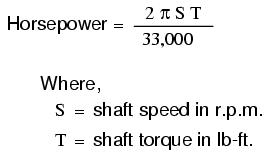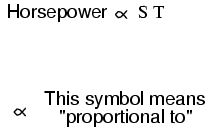# 2.3: Power in Electric Circuits

In addition to voltage and current, there is another measure of free electron activity in a circuit: power. First, we need to understand just what power is before we analyze it in any circuits.

Power is a measure of how much work can be performed in a given amount of time. Work is generally defined in terms of the lifting of a weight against the pull of gravity. The heavier the weight and/or the higher it is lifted, the more work has been done. Power is a measure of how rapidly a standard amount of work is done.

For American automobiles, engine power is rated in a unit called “horsepower,” invented initially as a way for steam engine manufacturers to quantify the working ability of their machines in terms of the most common power source of their day: horses. One horsepower is defined in British units as 550 ft-lbs of work per second of time. The power of a car’s engine won’t indicate how tall of a hill it can climb or how much weight it can tow, but it will indicate how fast it can climb a specific hill or tow a specific weight.

The power of a mechanical engine is a function of both the engine’s speed and its torque provided at the output shaft. Speed of an engine’s output shaft is measured in revolutions per minute, or RPM. Torque is the amount of twisting force produced by the engine, and it is usually measured in pound-feet, or lb-ft (not to be confused with foot-pounds or ft-lbs, which is the unit for work). Neither speed nor torque alone is a measure of an engine’s power.

A 100 horsepower diesel tractor engine will turn relatively slowly, but provide great amounts of torque. A 100 horsepower motorcycle engine will turn very fast, but provide relatively little torque. Both will produce 100 horsepower, but at different speeds and different torques. The equation for shaft horsepower is simple:Notice how there are only two variable terms on the right-hand side of the equation, S and T. All the other terms on that side are constant: 2, pi, and 33,000 are all constants (they do not change in value). The horsepower varies only with changes in speed and torque, nothing else. We can re-write the equation to show this relationship:Because the unit of the “horsepower” doesn’t coincide exactly with speed in revolutions per minute multiplied by torque in pound-feet, we can’t say that horsepower equals ST. However, they are proportionalto one another. As the mathematical product of ST changes, the value for horsepower will change by the same proportion.

In electric circuits, power is a function of both voltage and current. Not surprisingly, this relationship bears striking resemblance to the “proportional” horsepower formula above:In this case, however, power (P) is exactly equal to current (I) multiplied by voltage (E), rather than merely being proportional to IE. When using this formula, the unit of measurement for power is the watt, abbreviated with the letter “W.”

It must be understood that neither voltage nor current by themselves constitute power. Rather, power is the combination of both voltage and current in a circuit. Remember that voltage is the specific work (or potential energy) per unit charge, while current is the rate at which electric charges move through a conductor. Voltage (specific work) is analogous to the work done in lifting a weight against the pull of gravity. Current (rate) is analogous to the speed at which that weight is lifted. Together as a product (multiplication), voltage (work) and current (rate) constitute power.

Just as in the case of the diesel tractor engine and the motorcycle engine, a circuit with high voltage and low current may be dissipating the same amount of power as a circuit with low voltage and high current. Neither the amount of voltage alone nor the amount of current alone indicates the amount of power in an electric circuit.

In an open circuit, where voltage is present between the terminals of the source and there is zero current, there is zero power dissipated, no matter how great that voltage may be. Since P=IE and I=0 and anything multiplied by zero is zero, the power dissipated in any open circuit must be zero. Likewise, if we were to have a short circuit constructed of a loop of superconducting wire (absolutely zero resistance), we could have a condition of current in the loop with zero voltage, and likewise no power would be dissipated. Since P=IE and E=0 and anything multiplied by zero is zero, the power dissipated in a superconducting loop must be zero. (We’ll be exploring the topic of superconductivity in a later chapter).

Whether we measure power in the unit of “horsepower” or the unit of “watt,” we’re still talking about the same thing: how much work can be done in a given amount of time. The two units are not numerically equal, but they express the same kind of thing. In fact, European automobile manufacturers typically advertise their engine power in terms of kilowatts (kW), or thousands of watts, instead of horsepower! These two units of power are related to each other by a simple conversion formula:So, our 100 horsepower diesel and motorcycle engines could also be rated as “74570 watt” engines, or more properly, as “74.57 kilowatt” engines. In European engineering specifications, this rating would be the norm rather than the exception.

REVIEW:

• Power is the measure of how much work can be done in a given amount of time.
• Mechanical power is commonly measured (in America) in “horsepower.”
• Electrical power is almost always measured in “watts,” and it can be calculated by the formula P = IE.
• Electrical power is a product of both voltage and current, not either one separately.
• Horsepower and watts are merely two different units for describing the same kind of physical measurement, with 1 horsepower equaling 745.7 watts.